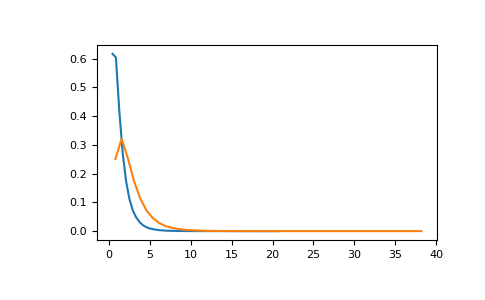#### Previous topic

numpy.random.RandomState.noncentral_chisquare

#### Next topic

numpy.random.RandomState.normal

# numpy.random.RandomState.noncentral_f¶

method

`RandomState.``noncentral_f`(dfnum, dfden, nonc, size=None)

Draw samples from the noncentral F distribution.

Samples are drawn from an F distribution with specified parameters, dfnum (degrees of freedom in numerator) and dfden (degrees of freedom in denominator), where both parameters > 1. nonc is the non-centrality parameter.

Note

New code should use the `noncentral_f` method of a `default_rng()` instance instead; see random-quick-start.

Parameters
dfnumfloat or array_like of floats

Numerator degrees of freedom, must be > 0.

Changed in version 1.14.0: Earlier NumPy versions required dfnum > 1.

dfdenfloat or array_like of floats

Denominator degrees of freedom, must be > 0.

noncfloat or array_like of floats

Non-centrality parameter, the sum of the squares of the numerator means, must be >= 0.

sizeint or tuple of ints, optional

Output shape. If the given shape is, e.g., `(m, n, k)`, then `m * n * k` samples are drawn. If size is `None` (default), a single value is returned if `dfnum`, `dfden`, and `nonc` are all scalars. Otherwise, `np.broadcast(dfnum, dfden, nonc).size` samples are drawn.

Returns
outndarray or scalar

Drawn samples from the parameterized noncentral Fisher distribution.

`Generator.noncentral_f`

which should be used for new code.

Notes

When calculating the power of an experiment (power = probability of rejecting the null hypothesis when a specific alternative is true) the non-central F statistic becomes important. When the null hypothesis is true, the F statistic follows a central F distribution. When the null hypothesis is not true, then it follows a non-central F statistic.

References

1

Weisstein, Eric W. “Noncentral F-Distribution.” From MathWorld–A Wolfram Web Resource. http://mathworld.wolfram.com/NoncentralF-Distribution.html

2

Wikipedia, “Noncentral F-distribution”, https://en.wikipedia.org/wiki/Noncentral_F-distribution

Examples

In a study, testing for a specific alternative to the null hypothesis requires use of the Noncentral F distribution. We need to calculate the area in the tail of the distribution that exceeds the value of the F distribution for the null hypothesis. We’ll plot the two probability distributions for comparison.

```>>> dfnum = 3 # between group deg of freedom
>>> dfden = 20 # within groups degrees of freedom
>>> nonc = 3.0
>>> nc_vals = np.random.noncentral_f(dfnum, dfden, nonc, 1000000)
>>> NF = np.histogram(nc_vals, bins=50, density=True)
>>> c_vals = np.random.f(dfnum, dfden, 1000000)
>>> F = np.histogram(c_vals, bins=50, density=True)
>>> import matplotlib.pyplot as plt
>>> plt.plot(F[1:], F)
>>> plt.plot(NF[1:], NF)
>>> plt.show()
```